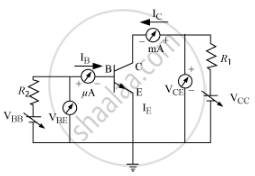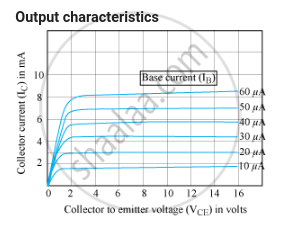# Answer the Following Question. Draw the Circuit Arrangement for Studying the Output Characteristics of an N-p-n Transistor in Ce Configuration. Explain How the Output Characteristics is Obtained. - Physics

Draw the circuit arrangement for studying the output characteristics of an n-p-n transistor in CE configuration. Explain how the output characteristics is obtained.

#### Solution

Circuit arrangement for studying the output characteristics of an n-p-n  transistor in CE configurationVariation of collector current IC with the collector − Emitter voltage VCE is called output characteristicsThe output characteristics describe the relationship between output current (IC) and output voltage (VCE).
First, draw a vertical line and a horizontal line. The vertical line represents the y-axis and horizontal line represents x-axis. The output current or collector current (IC) is taken along the y-axis (vertical line) and the output voltage (VCE) is taken along the x-axis (horizontal line).
To determine the output characteristics, the input current or base current IB is kept constant at 0 μA and the output voltage
VCE is increased from zero volts to different voltage levels. For each level of the output voltage, the corresponding output current (IC) is recorded.
A curve is then drawn between output current Iand output voltage VCE at constant input current IB (0 μA).

Concept: Junction Transistor - Basic Transistor Circuit Configurations and Transistor Characteristics
Is there an error in this question or solution?